Many DAX newbies use LASTDATE to search for the last date in a time period. Or they use NEXTDAY to retrieve the day after a given date. Although these functions do what they promise, they are not intended to be used in simple expressions. Instead, they are table functions designed to be used in time intelligence calculations. Using them the wrong way leads to inefficient code. Moreover, using these functions in ways they were not designed for is a telltale sign that the developer still does not grasp certain details of DAX.

In this article, we elaborate on the topic in order to understand what these time intelligence functions do; we also want to understand the reason why it is so easy to confuse them with simple math over dates. We want to elaborate on this topic through examples. Therefore, instead of starting with boring theory, we start by looking at a calculation that – although it works perfectly fine – is inherently wrong.

You want to compute the days included in a given selection, and build a report like the one below.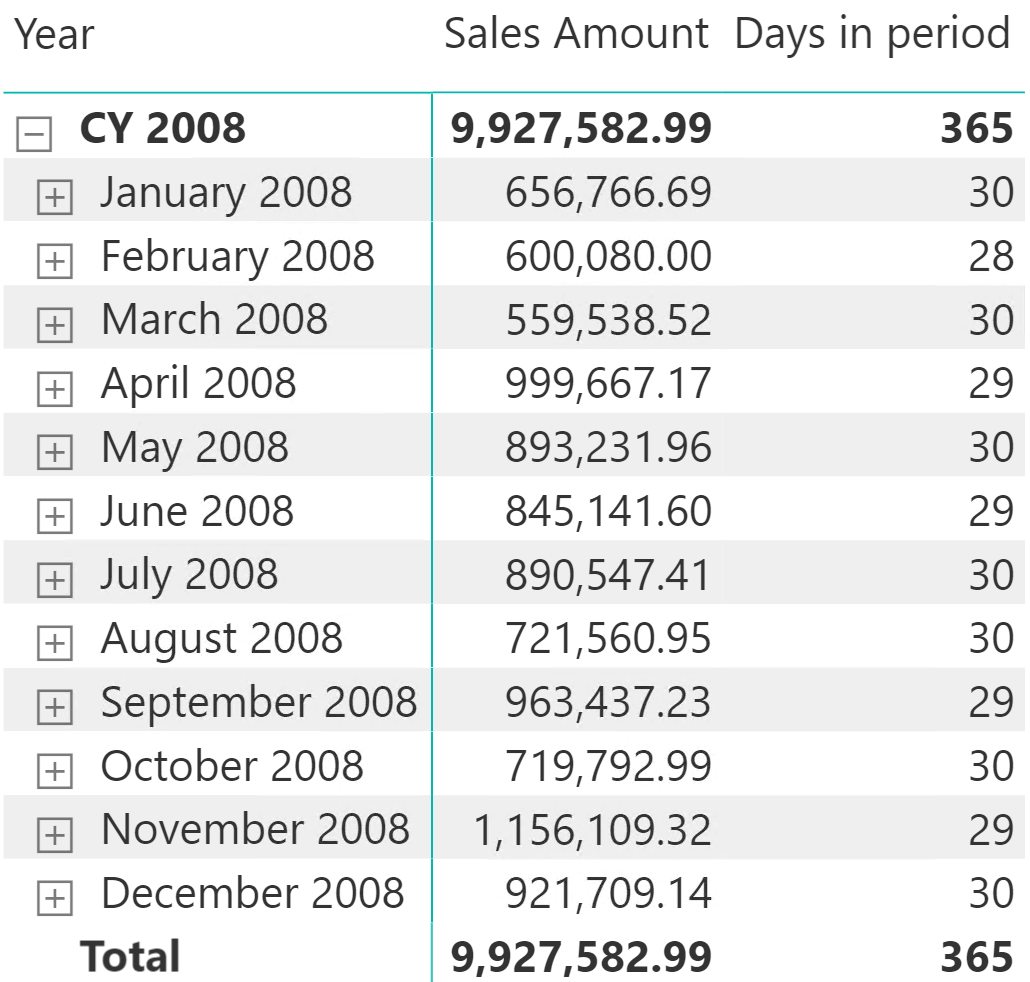Computing DaysInPeriod is straightforward: the number of days is the difference between the first and the last dates in the time period. DAX offers two functions: FIRSTDATE and LASTDATE, that seem like perfect candidates:

```Days in period :=
INT ( LASTDATE ( 'Date'[Date] ) - FIRSTDATE ( 'Date'[Date] ) )
```

This measure works fine and produces the right result. Therefore, we are happy! Right? Wrong. We are not happy because we are using LASTDATE to retrieve the value of the last visible date in a time period. LASTDATE performs exactly this job, but it returns a table containing that last date – not only the date. Let me repeat this: it does not return a date. It returns a table, containing the date.

The reason for this is that LASTDATE is a time intelligence function. Its main purpose is to be used as a filter argument in CALCULATE. CALCULATE filter arguments are tables. Therefore, for a function to be used in a measure like the following one, it needs to return a table:

```SalesOfLastDay =
CALCULATE (
[Sales Amount],
LASTDATE ( 'Date'[Date] )
)
```

You can double-check the result of LASTDATE by using DAX Studio. LASTDATE returns a table. This is why you can use it in an EVALUATE statement, which requires a table as its result.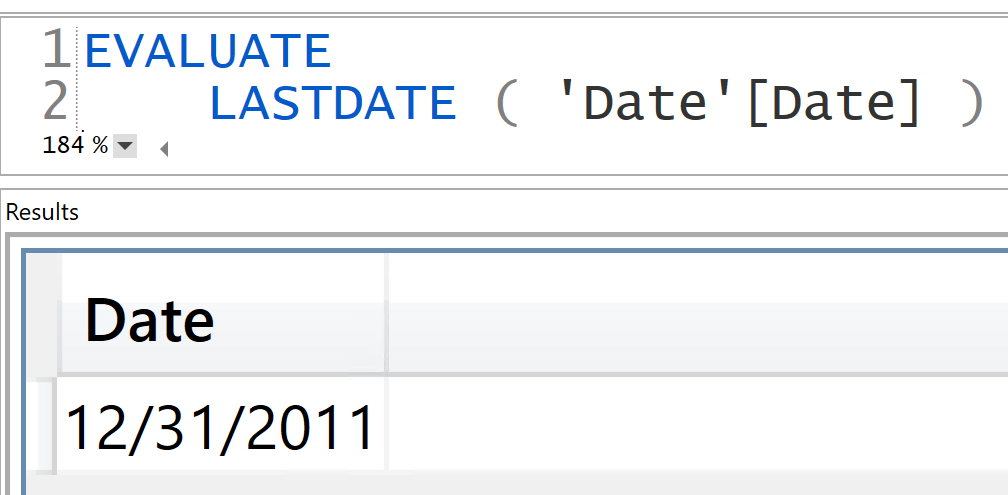As you see, the result is a table containing one column (Date) with the value of the last date.

In DAX, a table containing exactly one row and one column – the kind of result you would get from LASTDATE – can be used in lieu of the value inside. Indeed, a one-row-one-column table contains only one value. This is why DAX lets you automatically convert the table into a value. This is also the reason you can subtract two tables in our measure:

```Days in period :=
INT ( LASTDATE ( 'Date'[Date] ) - FIRSTDATE ( 'Date'[Date] ) )
```

Indeed, both LASTDATE and FIRSTDATE return tables. Because we are using a subtraction operator, DAX performs the conversion of the two tables into scalar values, and then computes the difference between the values contained inside the tables.

Although this behavior is transparent, it comes at a price. A better way to express the previous calculation is by using scalar functions, like MIN instead of FIRSTDATE and MAX instead of LASTDATE. MIN and MAX do not return tables: they return the values of the first and last dates. Therefore, a better formulation of the measure is the following:

```Days in period MIN MAX :=
INT ( MAX ( 'Date'[Date] ) - MIN ( 'Date'[Date] ) )
```

Again, you can double-check the result of MIN and MAX by using DAX Studio. If you try to use MAX instead of LASTDATE as the result of an EVALUATE statement, you obtain an error.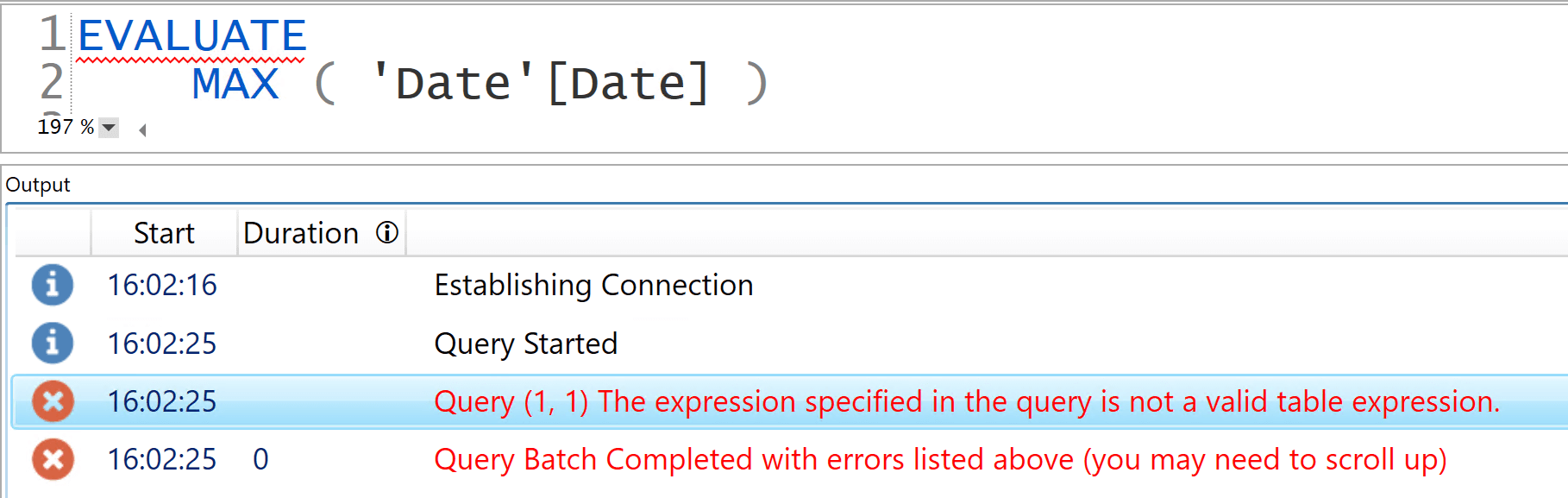In order to obtain a result with EVALUATE, you need to build a table containing the maximum date. You do this for example with a table constructor.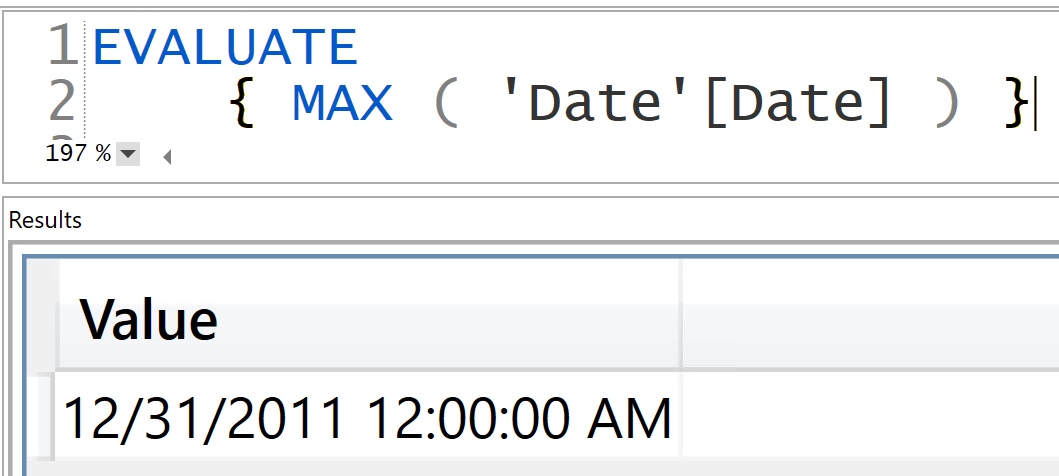As we said earlier, DAX automatically converts a table with one row and one column into a value. But this behavior comes at a price. Moreover, LASTDATE and FIRSTDATE both perform a context transition before finding the first and last date. This behavior is not affecting our simple example, but in more complex scenarios the performance might be very poor just because of this aspect.

This article is DAX 101; therefore, it should end here. But of course we cannot help but give out more details for the most curious among you. How can you check the difference in behavior between the two versions of our formula?

By using DAX Studio you can analyze the server timings of this query:

```--
--    This version uses FIRSTDATE and LASTDATE
--
EVALUATE
SUMMARIZECOLUMNS (
'Date'[Year Month],
"Days in period", [Days in period]
)
```

Despite being extremely fast, you can see from the server timings that the engine had to materialize the Date table twice: once for the Date[Date] column and once for the two columns Date[Date] and Date[Calendar Year Month], generating two data caches with 2,556 rows. The Formula Engine (FE) subsequently scans these data caches to compute the required result.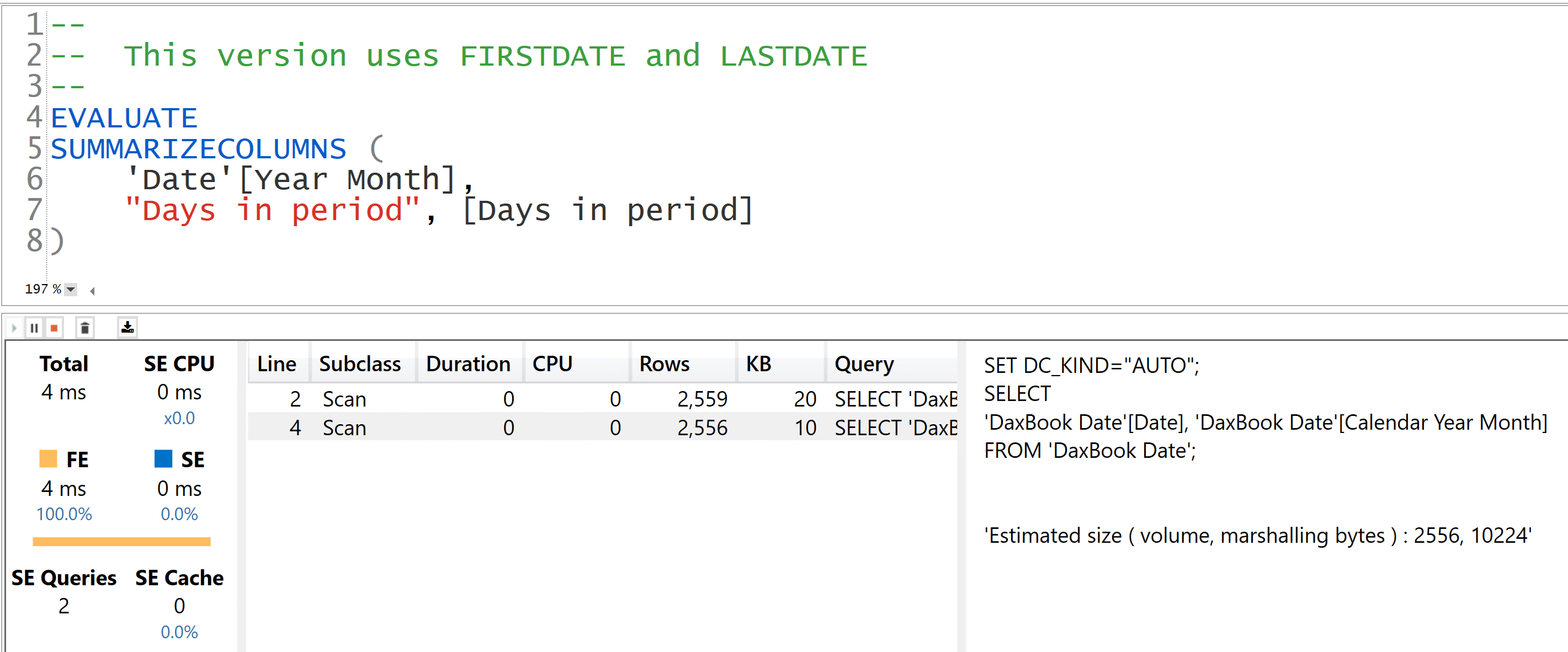With such a simple calculation, the query is not faster when it uses the optimized version, Days in period MIN MAX. Still, it is much better in terms of materialization because the entire calculation is pushed down to the Storage Engine (SE) which generates a single data cache with 87 rows: the same number of rows as the query result. Therefore, the Days in period MIN MAX measure produces an optimal materialization with the full computation executed by the SE. On larger models, or in more complex scenarios, this small difference in materialization might have a huge impact.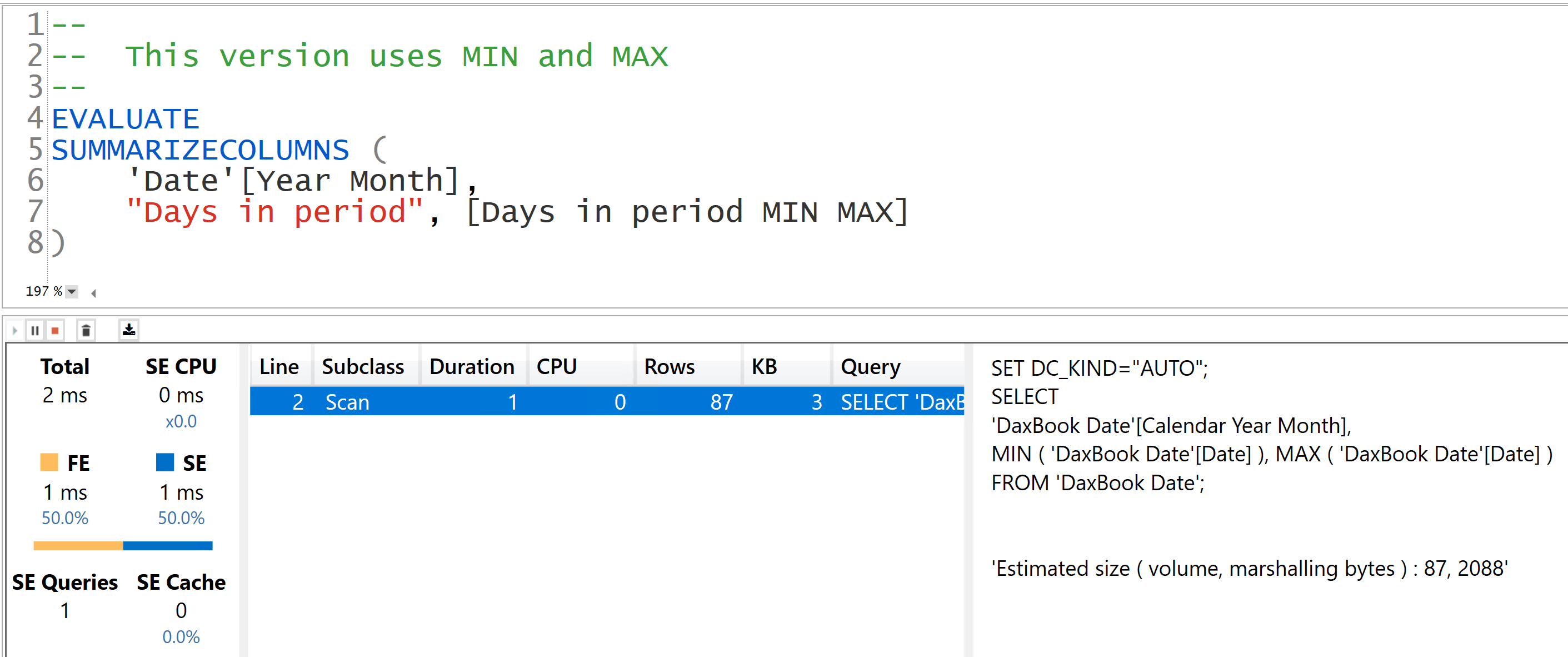Be mindful that most time intelligence functions (like FIRSTDATE, LASTDATE, NEXTDAY, PREVIOUSDAY…) display the same behavior: they return a table that can be automatically converted into a scalar value. But the conversion comes at a price that is not worth paying. It is always better to ask for exactly what you need: if you need a scalar value, use a scalar function. Use table functions only when you need a table as the result.

Using LASTDATE instead of MAX is not necessarily killing the performance of your calculations. It is weighing it down, which is not always noticeable with modern computers. That said, if you still confuse table functions with scalar functions, this is a symptom that there are still some details in the DAX language that you are not grasping. By paying attention to these details, you will become a better DAX developer!

LASTDATEContext transition

Returns last non blank date.

`LASTDATE ( <Dates> )`

NEXTDAYContext transition

Returns a next day.

`NEXTDAY ( <Dates> )`

FIRSTDATEContext transition

Returns first non blank date.

`FIRSTDATE ( <Dates> )`

CALCULATEContext transition

Evaluates an expression in a context modified by filters.

`CALCULATE ( <Expression> [, <Filter> [, <Filter> [, … ] ] ] )`

MINReturns the smallest value in a column, or the smaller value between two scalar expressions. Ignores logical values. Strings are compared according to alphabetical order.

`MIN ( <ColumnNameOrScalar1> [, <Scalar2>] )`

MAXReturns the largest value in a column, or the larger value between two scalar expressions. Ignores logical values. Strings are compared according to alphabetical order.

`MAX ( <ColumnNameOrScalar1> [, <Scalar2>] )`

PREVIOUSDAYContext transition

Returns a previous day.

`PREVIOUSDAY ( <Dates> )`